# A MATHEMATICAL MODEL OF GRADINGS

## 1 - A straight line

On the first page of this project the question was asked whether decline of performances of masters could be linear. The graphs of all masters records show that a straight line is a good approximation of decline during a big part of life. A straight line is the simplest graph and is taken as first approximation of performance decline and as first approximation of age gradings.
It would be strange when decline really should be linear, there are a lot of biological processes that change with age, starting at different ages and with different speed. But as long as it isn't clear which more complicated curves form a better model the simplest model is taken as first step.
A real model isn't developed here, which should be based on properties of biological processes. What I am going to do is not completely data driven either, as is the case with existing age gradings. Mathematical uniformity plays a role, mathematical simplicity too and sometimes parameters are influenced by the paramaters of neighbouring disciplines.

As test case triple jump of the men is taken, which clearly shows linearity: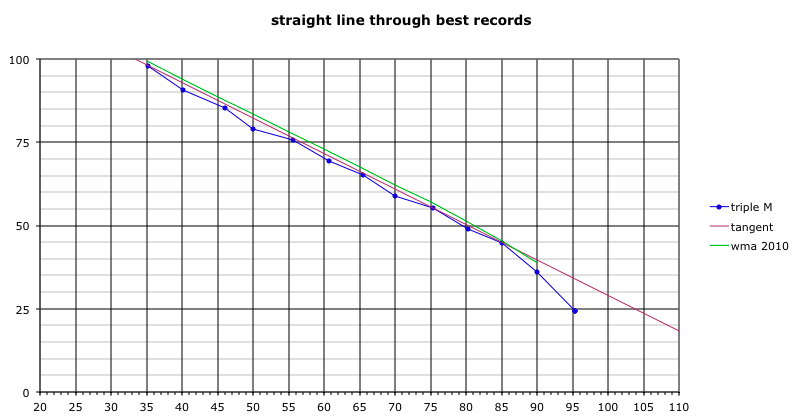Age in years is on the horizontal axis, percentage is on the vertical axis. The blue line connects all age records (five year age groups). The purple line is a line through two records such that all other records are below this line, the green line shows all performances that count as 100% in the current age grading of system 2010.
Note: for the rest of this page older data (early 2011) are used, with sometimes zigzagging record lines or two records per age class. For illustrating the model this is of no importance.

The graph below looks like the above one, the orange line shows the first model (in fact equal to the purple line). The heavy black line is the base line introduced on the previous page, an aid for making small differences more visible in coming graphs.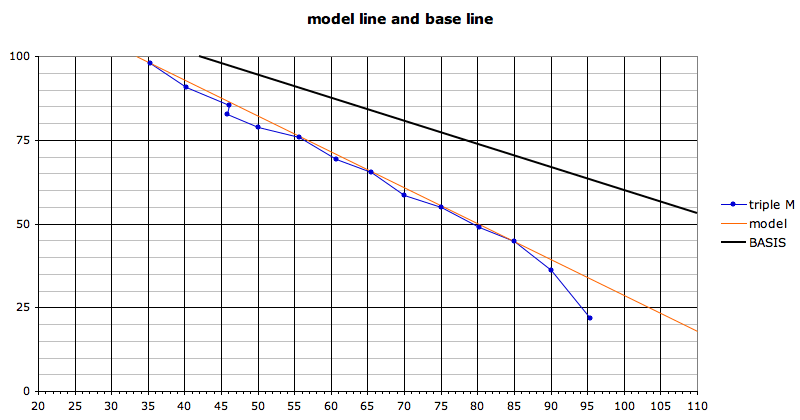Below a graph with enlarged differences, blue again are age records, orange is the model. The model goes through two records (M35 and M85) while three other records nearly are on the orange line (M55, M65 and M75).For those liking formulae: a straight line can be written in several forms, but is always determined by only two parameters. I give a form with parameters that make sense for masters athletics.
D = age when decline sets in, here 33.35 year
P = yearly decline percentage, here 1.07 %
The straight line is determined by two points (x1, y1) and (x2, y2) (x=age, y=percentage of record). Age is true age at the day of the record, not age in years.
In the background N plays a role in the calculation of the percentage, the norm that counts as 100%, here the OC world record of 18.29 meters.
The formula of the orange line now is: y = -Px + 100 + DP
(where x simply is age)

## 2 - Accelerated decline

In many disciplines the graphs show accelerated ageing in the older age groups. A curved line on top of the straight line will be able to follow the accelerated decline and I have chosen a very simple curve based on the series 0, 1, 3, 6, 10, 15, where the difference between terms grows with 1 at every step. In the model two extra parameters are needed:
A = age where acceleration sets in, here 86 years
F = a factor determining the strength of the acceleration, an arbitrary number, here 0.24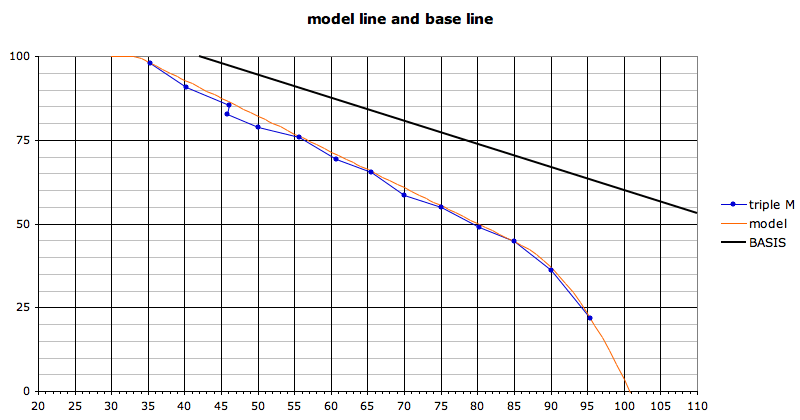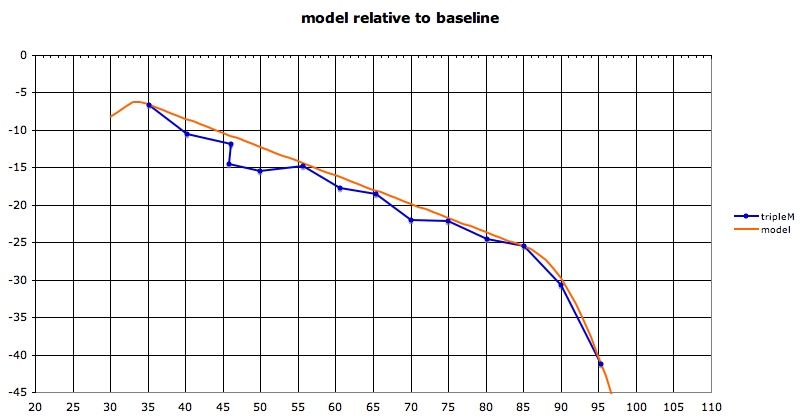## 3 - No straight line

Not always a straight line is the best first approximation of decline. Built in into the model is a possibility to transform the straight line to a concave or convex curve. An infinite number of curves exists, but here only a slight curvation is needed, the womens throws having the highest degree of curvation. The curve built in is part of a hyperbola (a hyperbola can be seen as a curved trasition between two straight lines). Two parameters are added:
C = amount of concaveness/convexness, roughly the number of percents the straight line is pressed up- or downwards in the middle of the graph.
W = a hyperbola can bend fast or slow, but in age gradings this has hardly any effect, therefore form factor W is kept constant.

## 4 - Offset

In the current system of age gradings (system 2010) the intention is that none of todays world records score 100%, as records will be improved in the future. The simplest way this can be done is to let all orange lines follow the records and shift them in the end one or two years to the right, the same shift in all disciplines. In this study it will not be applied.

## 5 - Tilt

It is very wel possible that strength of records of young and old athletes are not fully comparable. In the Netherlands in 2012 we started a proof in the club against club masters competition where all performances are age graded. When gradings work well young and old masters will get about the same number of points in the Hungarian table used in these competitions. Data are not yet sufficient to reach conclusions, but a first impression is that it doesn't work bad.
Nevertheless there is some rumor that old athletes are overrated. The data up to now do not exclude that possibility, maybe we can say more after another year.
But better would be to have lonigitudinal series (see page 1), long stretches of performances by the same athlete, two decades or even longer. After grading all these years there should not be a trend in the results.
To correct an eventual trend the straight line taken as basis in the model can be tilted. D remains the same but in higher ages the line goes up a bit. This possibility of tilting has been built in into the model and parameter T defines the strength of the effect.

## 6 - Start

The last two graphs show a sharp kink at the age where performances are not longer at OC level, here at age 33 and a bit. It is unlikely that you are performing at OC level this week and have to do with decline a week later, more likely is a period of several years of slightly declining physical possibilities before the 'normal' masters decline sets in. But there also is an effect working the other way: experience ever grows, both technically and in knowing how to train just hard enough to prevent injuries. This results in a long period of acting at OC level followed by a rather sudden onset of decline. In the model the kink could be smoothed, but the effect is small and it isn't built in.

## 7 - Dependence on the Open Class

Via parameter N everything is connected to the Open Class. Mistrusted OC records are not used in the calculations, but it is unknown whether remaining records can be trusted or not. The effect when another OC performance has been taken as N can be seen in the graphs below, with N=18.29 and N=17.29 (upper blue line). The blue masters record line then goes up, the effect being stronger in the younger age groups: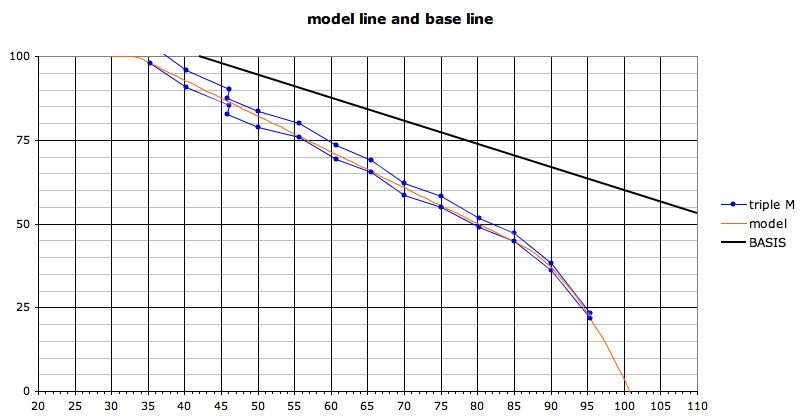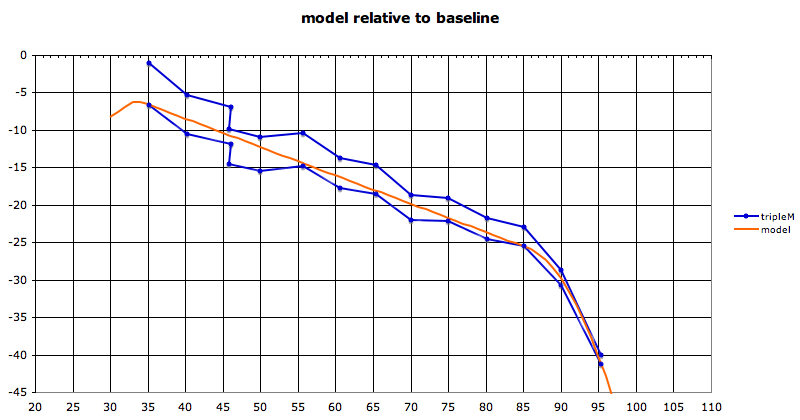Now it is time to test the model.